English

# How To: Identify and update calibration points that may not be following gap calibration rules

## Summary

In Esri Roads and Highways, it is possible to set calibration rules for how route edit operations impact route calibration when a gap exists in the route. There is a scenario described below in which these gap calibration rules may not be honored if you perform Retire Route or Reassign Route in the middle of a route. In this scenario, the measures on both the sides of the gap (upstream and downstream) remain unchanged as a result of the edit.

```Note:
Even though the issue is no longer present as of Esri Roads and Highways 10.5 Patch 1, it is necessary to determine if gap calibration rules have been followed for any editing performed in Esri Road and Highways 10.5 and earlier.
```

If contacting Esri Support, please refer to TFS 51854: Reversing then reassigning a middle portion of a route results in an incorrect gap calibration method being applied to the downstream portion of the route.

## Procedure

##### Gap Calibration issues when retiring in the middle of a route
1. The following screenshot shows calibration points (shown in pink) prior to a retirement in the middle of the route.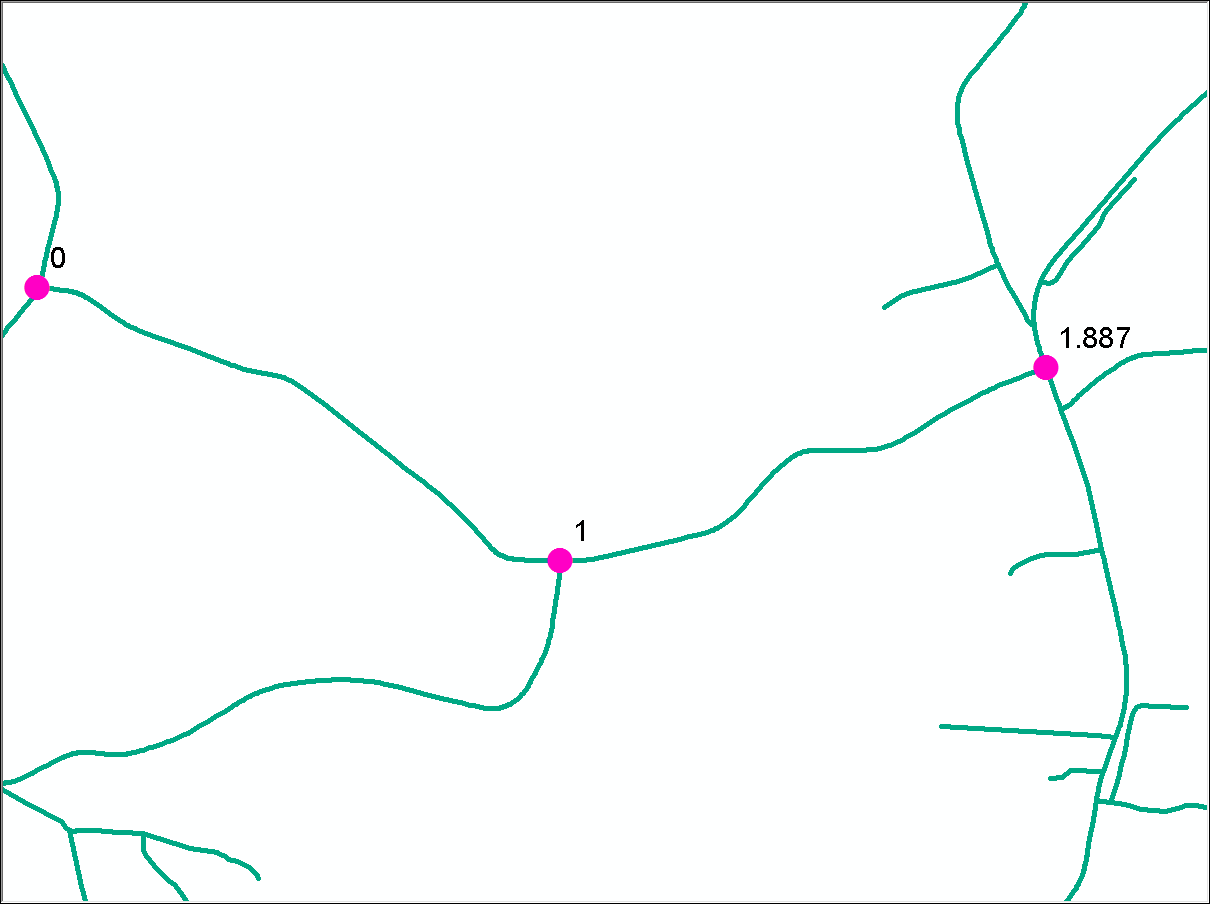1. Adding Euclidean Distance is set as the Gap Calibration rule in the LRS Network Properties for this LRS network.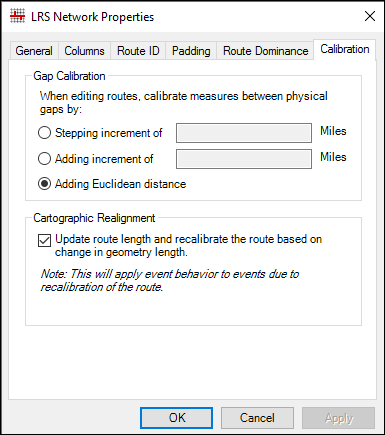1. These are the parameters used for the retirement. The portion of the route between the light pink and purple dots will be retired, from measure 1.1 miles to measure 1.3 miles.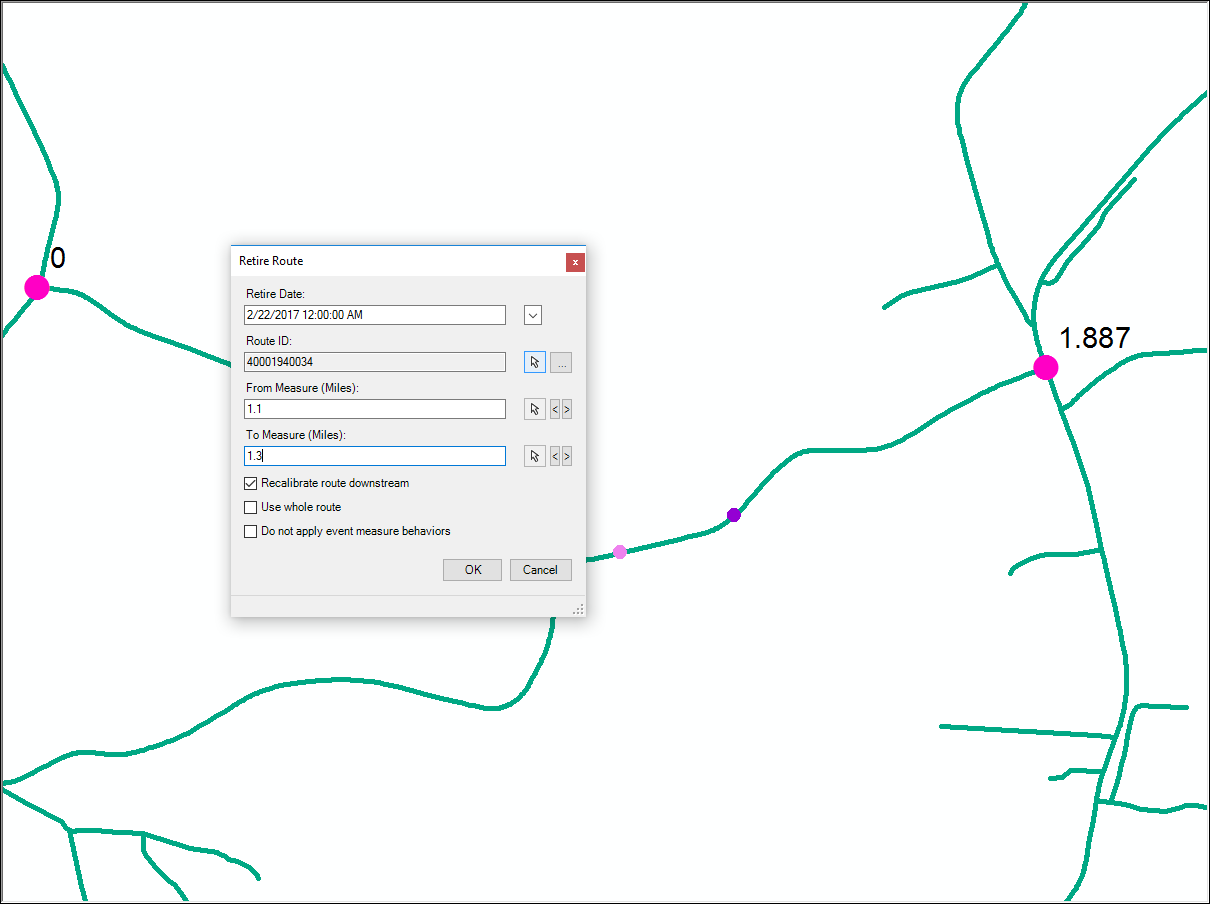1. This is the output after the Retire tool is run. Notice the gap in the middle of the route where the portion of the route has been retired.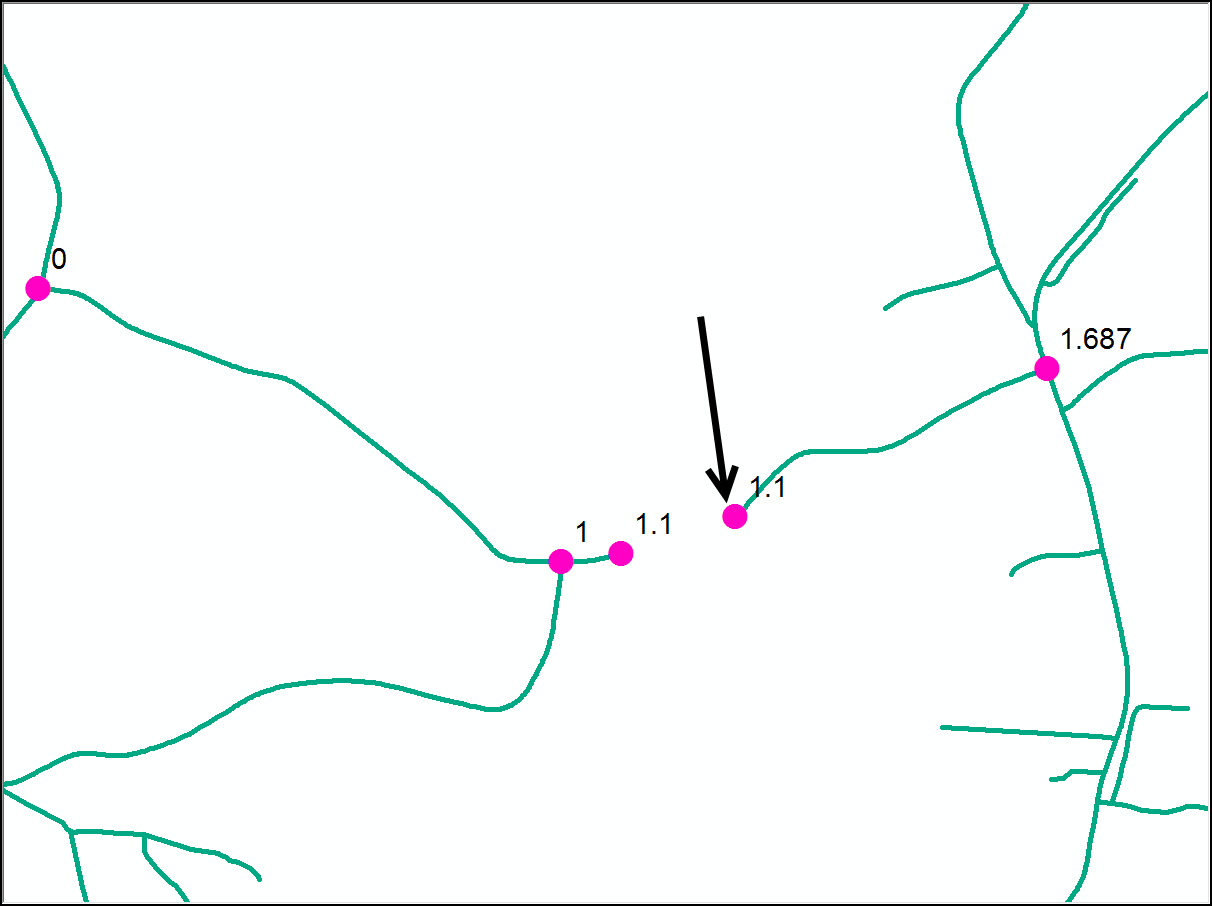1. The gap calibration rules mandate that the calibration point at the end of the gap (downstream of the gap, or on the right side of the gap in this example) must include the Euclidean distance between the start of the gap and end of the gap. In this case, from the start of the gap to the end of the gap has a Euclidean distance of 0.2 miles, and the calibration point at the end of the gap must have a measure of 1.3 miles. However, the calibration point at the end of the gap shows 1.1 miles.
##### How to identify calibration points that may not be following the gap calibration rules
1. Add the Calibration Point layer to ArcMap and verify the Esri Roads and Highways extension is active. In the below example, there are two calibration points shown with the measure of 0.5 that are located on either side of a gap. This gap was created by retiring a portion of the route in the middle of the route.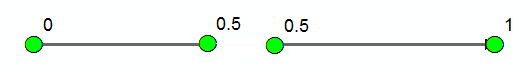1. Ensure the time filter is set to a valid date. For example: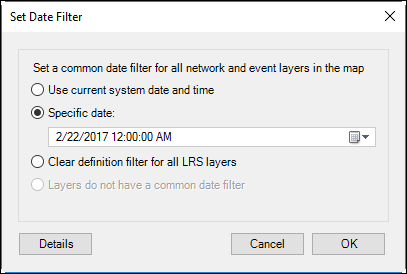1. Open the Frequency geoprocessing tool in the Analysis Toolbox> Statistics Toolset. Check the RouteId and Measure fields as the Frequency Field(s) parameter input as shown. The output can be to a scratch geodatabase.
```Note: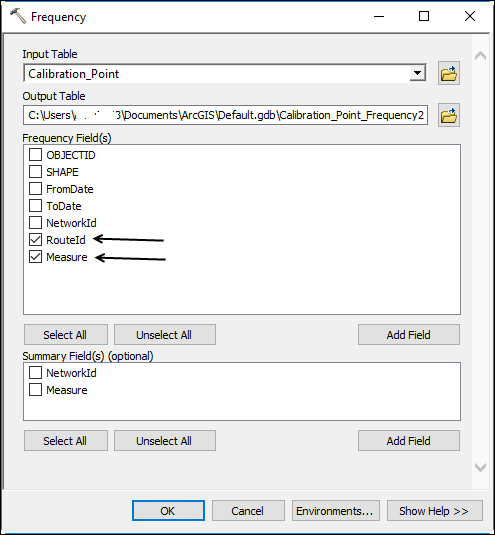1. Open the output table.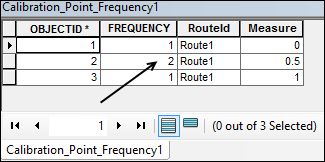1. In the output table, any RouteId and Measure combination with a Frequency value of less than 1 implies a location on that RouteId where more than one calibration point has the same value. As shown in the example, the measure 0.5 has a frequency of 2 for RouteId Route1. Verify in the figure provided above that the calibration points on either sides of the gap in fact have the same value of 0.5.

#### How to update the calibration points that may not be following gap calibration rules

1. Create a geodatabase version of your data and use the new version for performing this workflow.
2. After identifying the route locations where calibration points on both sides of a gap have the same value, use the Edit Calibration Point tool to edit the calibration point at the end of the gap (downstream of the gap). This sets the measure equal to the sum of the measure at the start of the gap plus the Euclidean distance spanning the gap. In the example, the calibration point is 1.3 (Calibration Point at the start of the gap is equal to 1.1; the Euclidean distance to span the gap from the start of gap to end of the gap is equal to .2; so 1.1 + .2 = 1.3 for the updated measure).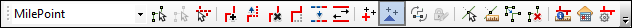1. Verify the Recalibrate downstream option is checked. This will add the delta (the change, or increase in measure in this scenario) to the calibration points downstream of the edit.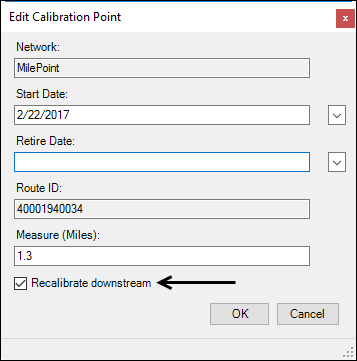1. As shown below, a delta of 0.2 is added to each calibration point along the route downstream of the gap.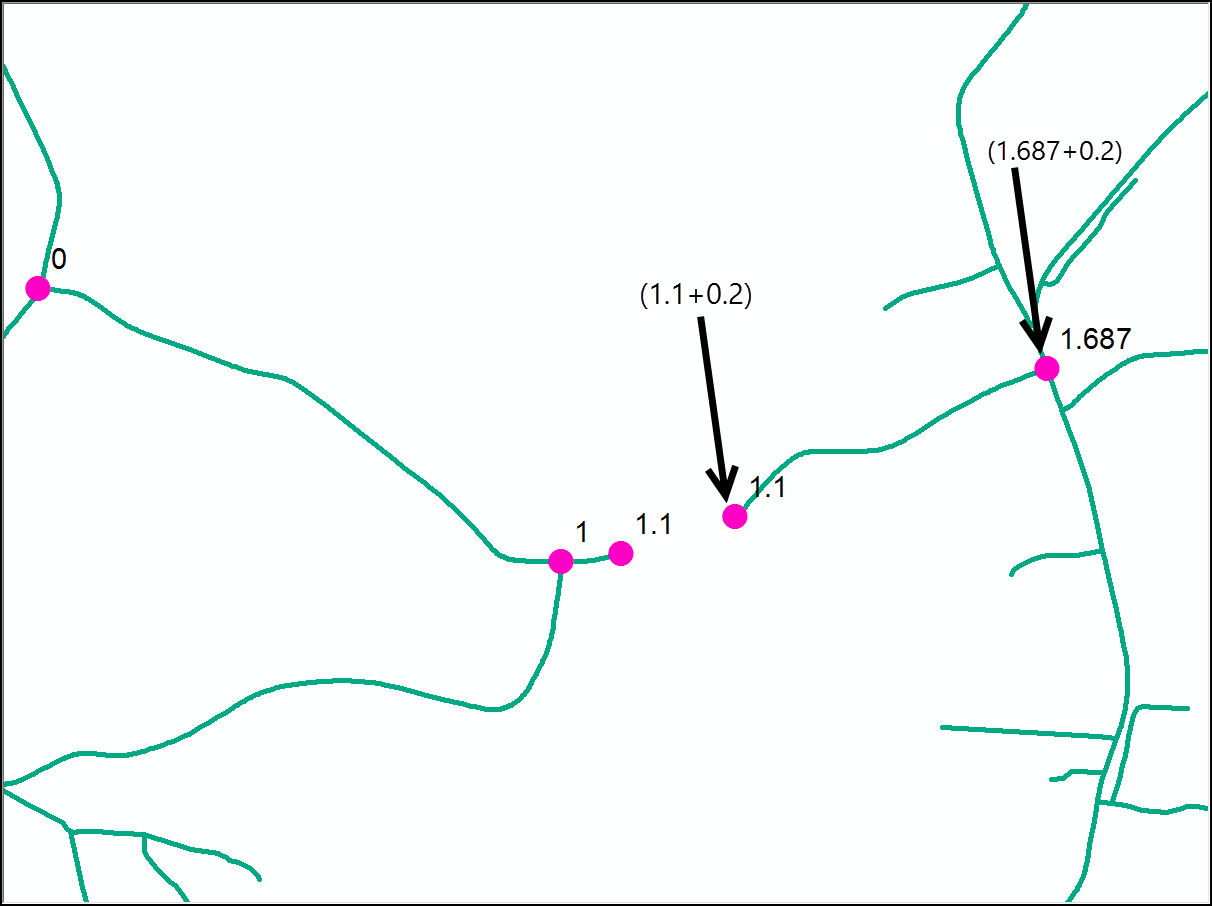1. Run the Apply Updates tool to update the events impacted by the calibration point edits.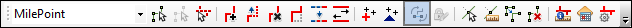1. Save the edits after confirming the changes are as desired. Use the Reconcile and Post operations to sync these changes to the parent version and continue with the content quality workflow.